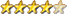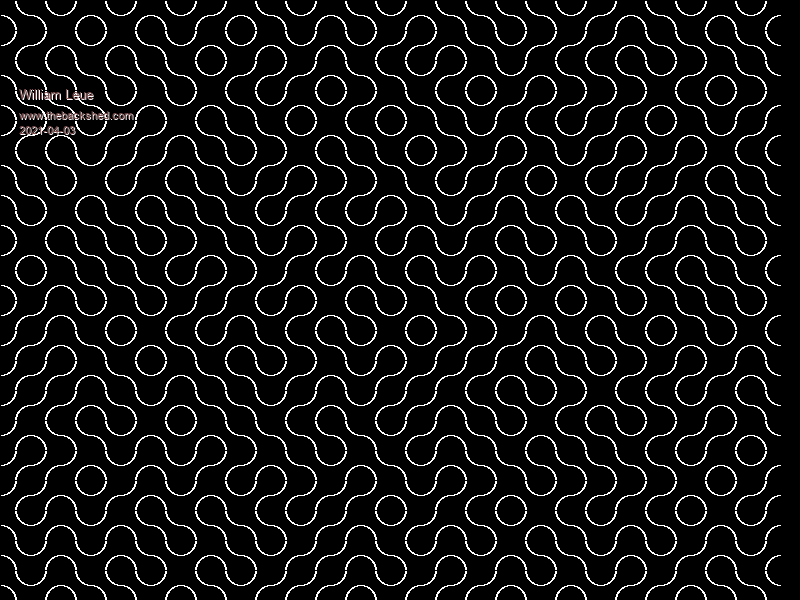Simple Maze using Truchet Tiles

Author Message
William Leue
GuruJoined: 03/07/2020
Location: United States
Posts: 365
 Posted: 03:14pm 02 Apr 2021

I'm tired of wrestling with the code for some of my more ambitious CMM2 projects, so here's a tiny little program that creates a sort-of maze using Truchet Tiles (see Wikipedia). It has a very close relationship to the famous one-line Commodore Pet maze generator known as '10Print'.

' Maze formed from Truchet Tiles
' This is basically the same algorithm as the famous
' Commodore Pet '10Print' one-line maze.
' Rev 1.0.0 William M Leue 4/1/2021
option default integer
option base 1

const TSIZE = 30

cls
ncols = MM.HRES\TSIZE
nrows = MM.VRES\TSIZE
for row = 1 to nrows
y = (row-1)*TSIZE
for col = 1 to ncols
x = (col-1)*TSIZE
DrawTruchetTile x, y, rnd()
next col
next row
end

sub DrawTruchetTile x, y, r as float
local cx, cy
if r < 0.5 then
cx = x : cy = y + TSIZE
arc cx, cy, TSIZE\2,, 0, 90
cx = x + TSIZE : cy = y
arc cx, cy, TSIZE\2,, 180, 270
else
cx = x: cy = y
arc cx, cy, TSIZE\2,, 90, 180
cx = x + TSIZE: cy = y + TSIZE
arc cx, cy, TSIZE\2,, -90, 0
end if
end sub